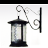# How to change Domain property in SpatialReferenceBuilder?

238
1
06-07-2019 11:18 AMby
New Contributor II

Domain is a read-only member of SpatialReferenceBuilder.  But I need to expand the coordinate system to fix out of bound during editing.  But I can not do so through Domain since it is read-only.  Is there a way to that?byEsri Contributor

Hi Fayu,

We will add a setter for the Domain property in the next release. In the meantime, you can change the xy-scale which will change the domain.

Here is an example:

``````const long suLimit = 9007199254740990;

// Default domain for Web Mercator is xmin = -20037700,
// ymin = -30241100, xmax = 900699887774.099, ymax = 900689684374.099
// with xyScale = 10000

// To change the domain change the xyScale. This will keep the same false origin,
// but xmax and ymax will change.
// Make the xyScale smaller => xmax, ymax are bigger.
// xyScale bigger => xmax, ymax are smaller.

SpatialReferenceBuilder builder = new SpatialReferenceBuilder(SpatialReferences.WebMercator);

// Suppose I know what I want my domain to be, then I have to figure out the scale.
// xyScale = suLimit / (xmax - xmin)
// assuming that xmax - xmin = ymax - ymin i.e. domain is a square.
// If xmax - xmin != ymax - ymin, take the larger of the two.

// Expand the default domain
double xMin = -20037700;
double xMax = 9007179217040.99;
double yMin = -30241100;
double yMax = 9007169013640.99;

double xyScale = suLimit / Math.Max(xMax - xMin, yMax - yMin); // xyScale = 1000
builder.XYScale = xyScale;

Envelope domain = builder.Domain;
Console.Out.WriteLine(\$"xMin = {domain.XMin}");
Console.Out.WriteLine(\$"xMax = {domain.XMax}");
Console.Out.WriteLine(\$"yMin = {domain.YMin}");
Console.Out.WriteLine(\$"yMax = {domain.YMax}");‍‍‍‍‍‍‍‍‍‍‍‍‍‍‍‍‍‍‍‍‍‍‍‍‍‍‍‍‍‍‍‍‍‍‍‍‍‍‍‍‍‍‍‍‍‍‍‍‍‍‍‍‍‍‍‍‍‍‍‍‍‍‍‍‍‍‍‍‍‍‍‍‍‍‍‍‍‍‍‍‍‍‍‍‍‍‍‍‍‍‍‍‍‍‍‍‍‍‍‍‍‍‍‍‍‍‍‍‍‍‍‍‍‍‍‍‍‍‍‍‍‍‍``````

Let me know if you have any questions.

Annette